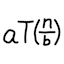#Master theorem solver (JavaScript)

In the study of complexity theory in computer science, analyzing the asymptotic run time of a recursive algorithm typically requires you to solve a recurrence relation. This JavaScript program automatically solves your given recurrence relation by applying the versatile master theorem (a.k.a. master method). However, it only supports functions that are polynomial or polylogarithmic. (The source code is available for viewing.)

## Program

### Input

Format: $$T(n) \: = \: a \: T(n \, / \, b) \, + \, Θ(n^k \, (\log n)^i)$$.

 $$a$$: $$b$$: $$k$$: $$i$$: (usually 0)

Recurrence:
Solution:

## Examples

Click on an example to run the numbers in the calculator above: